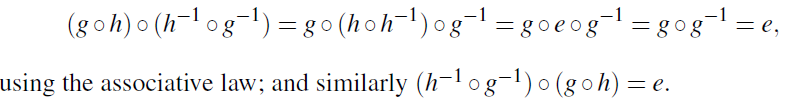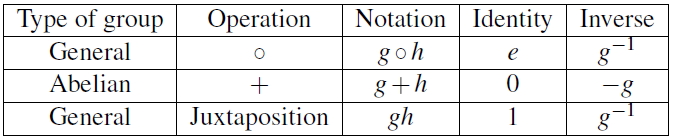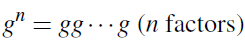Properties of groups: Some of these properties will look very familiar, since they are similar to what we saw for rings.

Uniqueness of identity element: The identity element of a group is unique. For suppose that there are two identity elements, say e1 and e2. (This means that g  e1 = e1  g = g for all g, and also g  e2 = e2  g = g for all g.) Then e1 = e1 . e2 = e2.

Uniqueness of inverse: The inverse of a group element g is unique. For suppose that h and k are both additive inverses of g. (This means that gh = hg = e and gk = k g = e – we know now that there is a unique identity element e). Then
h = h . e = h . (g . k) = (h . g) . k = e . k = k, where we use the associative law in the third step. We denote the inverse of g by g−1.

Composing more than two elements: We showed in Proposition 2.6 that, as long as the associative law holds, the result of composing any number of elements is independent of the way that the product is bracketed: for example, a  ((b  c)  d) = (a  b)  (c  d). Since the associative law holds in a group, we have:

Proposition 3.3 Let g1, . . . ,gn be elements of a group G. Then the composition g1  g2  · · ·  gn is well-defined, independent of the way it is bracketed. Cancellation laws

Proposition 3.4 In a group G, if ag=bg, then a=b. Similarly, if ga=gb, then a = b.
Proof Suppose that a  g = b. g, and let h = g−1. Then a = a . e = a . (g . h) = (a . g) . h = (b . g)  h = b . (g . h) = b . e = b. The other law is proved similarly. These facts are the cancellation laws.
Proposition 3.5 The inverse of g  h is h−1  g−1.
ProofNotation: The notation g.h for the group operation is a bit cumbersome, and we now change things.
If we are only interested in Abelian groups, we use as the symbol for the group operation, 0 for the group identity, and −g for the inverse of g. This agrees with the additive notation in a ring. Indeed, the additive group of a ring is an Abelian group, and every Abelian group is the additive group of a ring. [To see this, take the group operation as addition, and construct the zero ring: all products are zero.]
For general groups which may not be Abelian, we use juxtaposition for the group operation, 1 for the identity, and g−1 for the inverse of g. (This is like multiplicative notation in a ring, but it is not true that every group is the group of units in some ring!!)
This table gives the correspondences.For the rest of this course, our notation for the group operation will be juxtaposition.

Order: The term order has two quite different meanings in group theory: be careful not to confuse them. In the next chapter we will see that there is a close relationship between the two meanings.
The order of a group is the number of elements of the group. It may be finite (in which case it is a positive integer), or infinite.

To define the second kind of order, we introduce the notation gn. This means the result of composing n factors g together:More formally, g0 = 1, and for any positive integer n, gn = g · gn−1. The order of an element g in a group is defined as follows:
• If gn = 1 for some positive integer n, then the smallest such n is called the order of g.
• If no such n exists, we say that g has infinite order.
Thus, the identity element always has order 1. If an element g has order 2, then it is equal to its inverse (for g2 = 1 = gg−1 implies g = g−1 by the Cancellation
Law.)
Consider the additive group of the ring Z. (Recall that the operation is and the zero element is 0; so, instead of gn we write n · g, and the order is the smallest positive n such that n · g = 0, or is infinite if no such n exists.) The element 1 has infinite order, since there is no positive integer n such that n · 1 = 0. In the first group in our two examples above of Cayley tables, the elements x and z have order 4 (we have x2 = y, x3 = z, x4 = e which is the identity element), while y has order 2. In the second group, all of a,b,c have order 2.
Symmetric groups: Let X be any set. A permutation of X is a function g : X !X which is one-toone and onto, that is, a bijection from X to X.
Let Sn be the set of all permutations of the set {1, . . . ,n}. We have
|Sn| = n! = n(n−1)(n−2) · · ·1.
For consider the two-line representation. The top row is (12 . . . n). The bottom row consists of the same numbers in any order. Thus there are n possibilities for
the first entry in the bottom row; n−1 possibilities for the second (anything except the first), n−2 possibilities for the third; and so on.
Now we define an operation on permutations as follows. If g is a permutation, denote the image of the element x ∈ {1, . . . ,n} by xg. (As with homomorphisms, we write the function on the right of its input.) Now if g and h are two permutations, their composition g1g2 is defined by
x(gh) = (xg)h for all x ∈ {1, . . . ,n}.

In other words the rule is “apply g, then h”.
For example, if g is the permutation (1,3,5)(2,4)(6) in our above example, and h = (1,2,3,4,5,6), then gh = (1,4,3,6)(2,5). You are strongly urged to practice composing permutations given in cycle form!